``````library(Sleuth3)
library(ggplot2)
source(url("http://stat512.cwick.co.nz/code/stat_qqline.r"))
``````
``````## Loading required package: proto
``````
``````
# look at the first few entries
``````
``````##   Age Rate
## 1 0.1   53
## 2 0.2   38
## 3 0.3   58
## 4 0.3   52
## 5 0.3   42
## 6 0.4   62
``````
``````
# Fit the model
fit <- lm(Rate ~ Age, data = ex0824)

# Look at the TWO residual plots to assess linearity and constant spread
qplot(.fitted, .resid, data = fit) + geom_smooth()  #Residuals Vs. fitted values
``````
``````## geom_smooth: method="auto" and size of largest group is <1000, so using loess. Use 'method = x' to change the smoothing method.
``````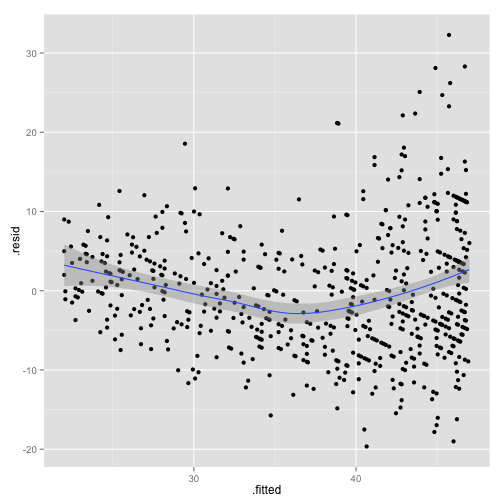``````qplot(Age, .resid, data = fit) + geom_smooth()  #Residuals Vs. Age (explanatory variable)
``````
``````## geom_smooth: method="auto" and size of largest group is <1000, so using loess. Use 'method = x' to change the smoothing method.
``````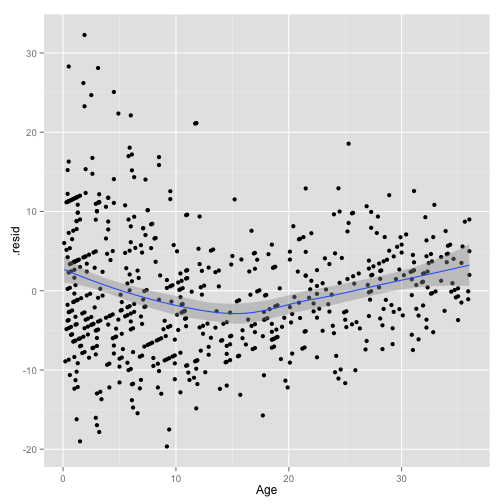From these two plots it definately looks like like the constant variance assumption is violated. Exploratory analysis indicates that as age increases the standard deviation (or variance or spread) decreases. While less evident it also appears the linearity assumption is also violated.

``````# Let's also check the normality
qplot(sample = .resid, data = fit) + stat_qqline()
``````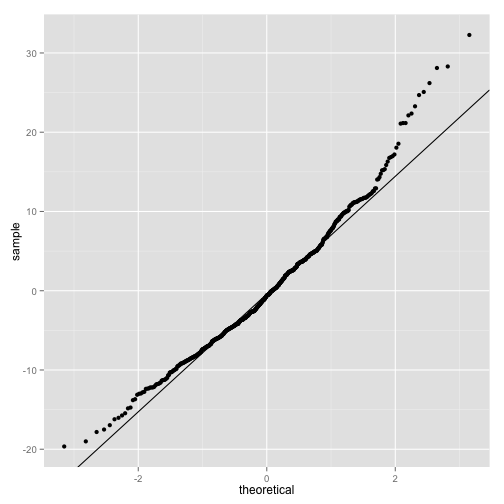From this plot it appears there are some outliers on the right. So our assumption about normality may not be that great. How will this affect our results? Let's see what the fabulous log transform does here

``````fit <- lm(log(Rate) ~ Age, data = ex0824)

# Look at the TWO residual plots to assess linearity and constant spread
qplot(.fitted, .resid, data = fit) + geom_smooth()  #Residuals Vs. fitted values
``````
``````## geom_smooth: method="auto" and size of largest group is <1000, so using loess. Use 'method = x' to change the smoothing method.
``````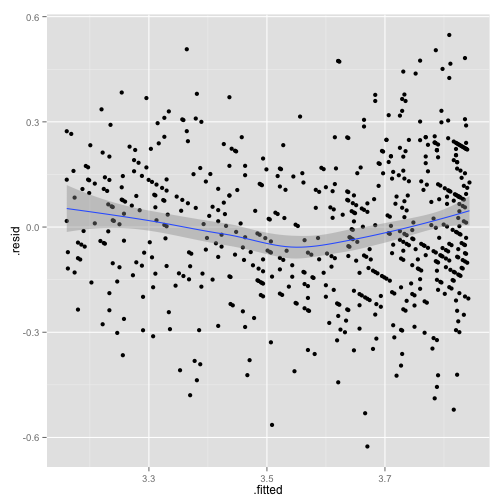``````qplot(Age, .resid, data = fit) + geom_smooth()  #Residuals Vs. Age (explanatory variable)
``````
``````## geom_smooth: method="auto" and size of largest group is <1000, so using loess. Use 'method = x' to change the smoothing method.
``````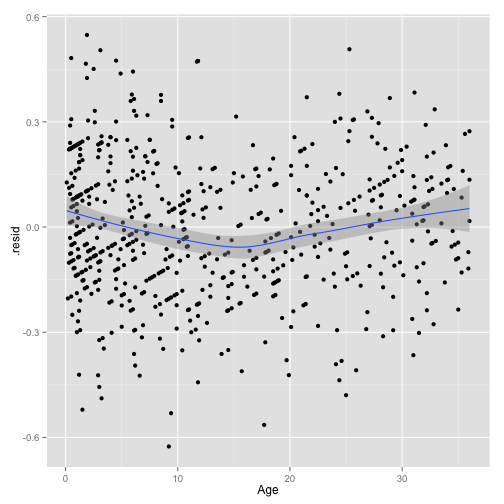``````
# Let's also check the normality
qplot(sample = .resid, data = fit) + stat_qqline()
``````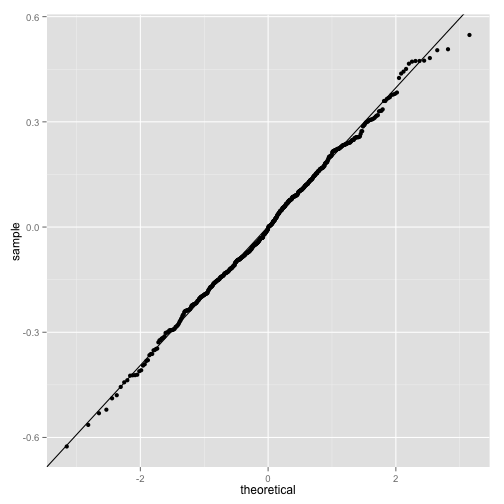This time it appears to me that all of our assumptions are met. So now how about we look at the interpretation of the slope. Remeber we log transformed so first let us find the estimate and back transform.

``````summary(fit)  #This tells us the estimate of the slope is -0.019
``````
``````##
## Call:
## lm(formula = log(Rate) ~ Age, data = ex0824)
##
## Residuals:
##    Min     1Q Median     3Q    Max
## -0.626 -0.132 -0.004  0.135  0.548
##
## Coefficients:
##              Estimate Std. Error t value Pr(>|t|)
## (Intercept)  3.845119   0.012628   304.5   <2e-16 ***
## Age         -0.019009   0.000736   -25.8   <2e-16 ***
## ---
## Signif. codes:  0 '***' 0.001 '**' 0.01 '*' 0.05 '.' 0.1 ' ' 1
##
## Residual standard error: 0.196 on 616 degrees of freedom
## Multiple R-squared:  0.52,   Adjusted R-squared:  0.519
## F-statistic:  668 on 1 and 616 DF,  p-value: <2e-16
``````
``````exp(-0.019)  # this value is equal to 0.9812
``````
``````##  0.9812
``````
``````exp(-0.019 - 1.96 * 0.0007357)
``````
``````##  0.9798
``````
``````exp(-0.019 + 1.96 * 0.0007357)  #This is the 95% CI and the values are 0.9798 and 0.9826
``````
``````##  0.9826
``````

Sample evidence indicates that an increase in age of one year is associated with a multiplicative change in the median respiratory rate of 0.9825. (corresponding 95% Confidence interval 0.9798 and 0.9826). So in regualar english “As age increases by 1 year the median respiratory rate will be about 2% less.”